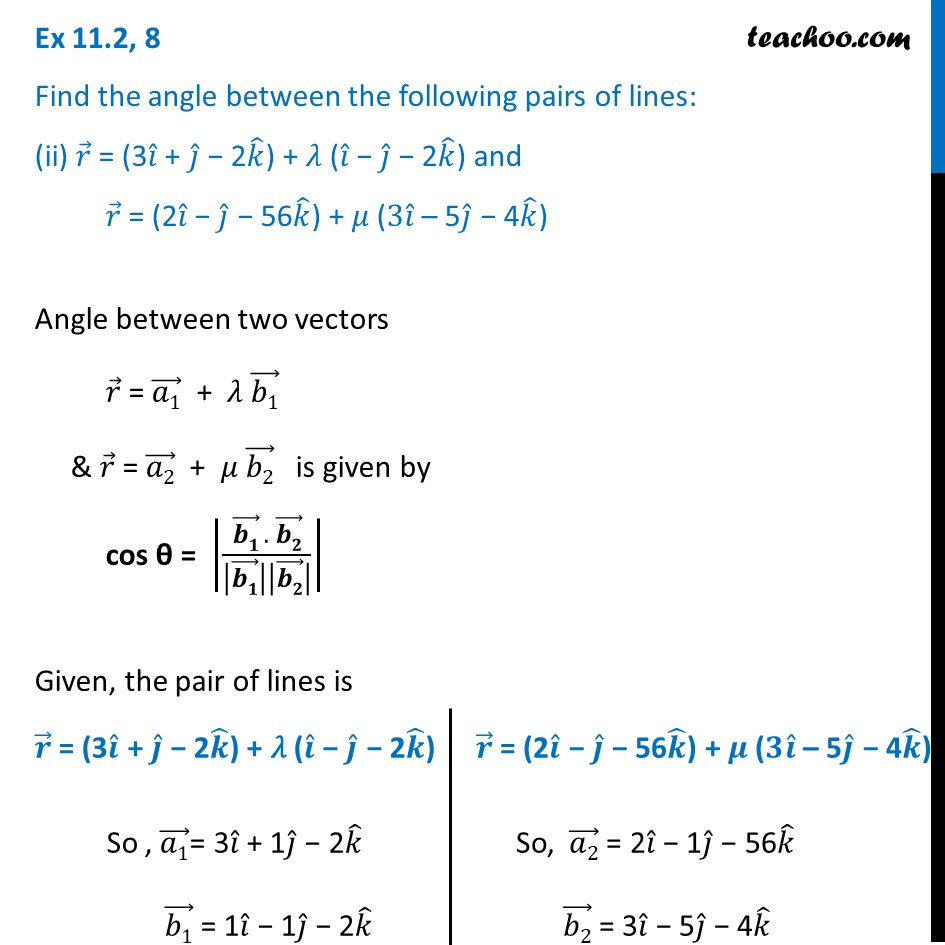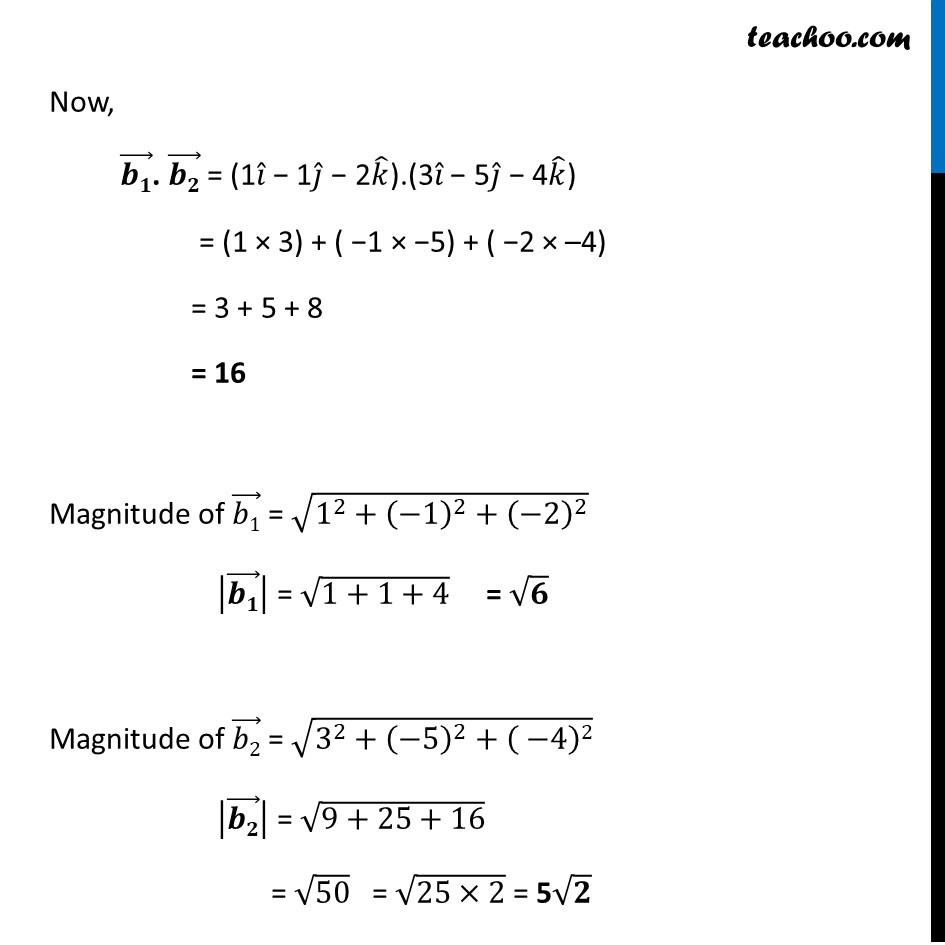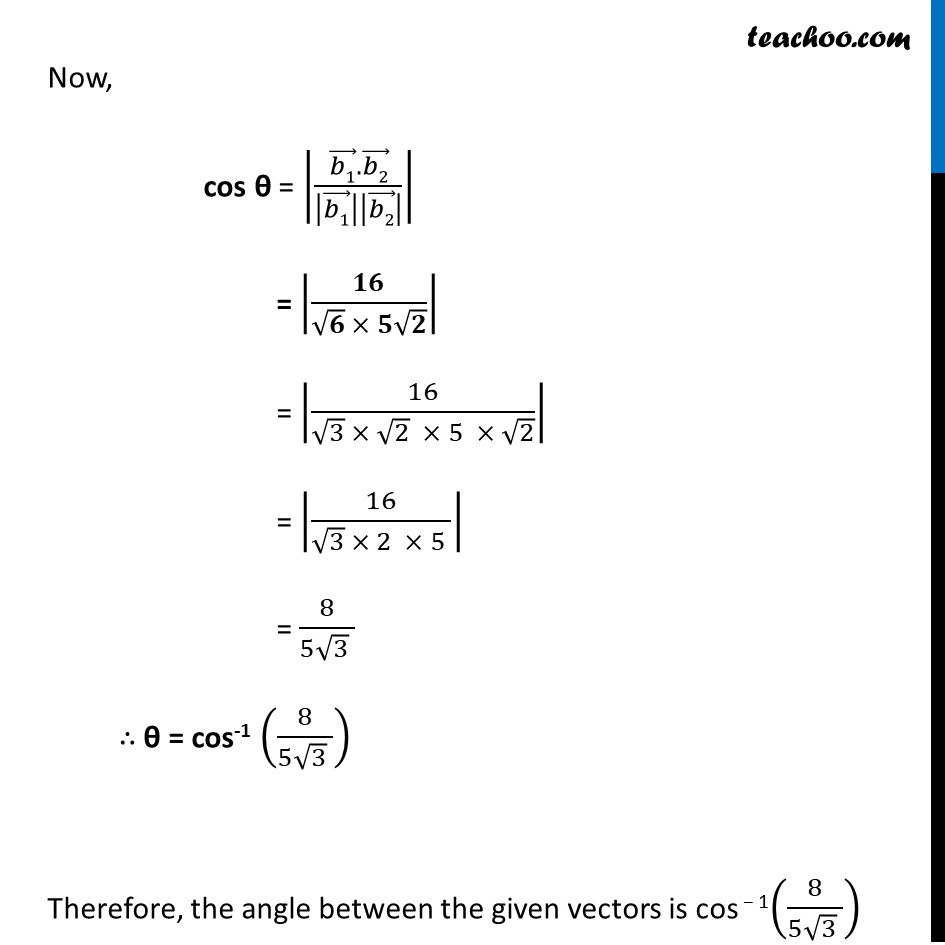Ex 11.2

Chapter 11 Class 12 Three Dimensional Geometry
Serial order wiseLearn in your speed, with individual attention - Teachoo Maths 1-on-1 Class

### Transcript

Ex 11.2, 8 Find the angle between the following pairs of lines: (ii) 𝑟 ⃗ = (3𝑖 ̂ + 𝑗 ̂ − 2𝑘 ̂) + 𝜆 (𝑖 ̂ − 𝑗 ̂ − 2𝑘 ̂) and 𝑟 ⃗ = (2𝑖 ̂ − 𝑗 ̂ − 56𝑘 ̂) + 𝜇 (3𝑖 ̂ – 5𝑗 ̂ − 4𝑘 ̂)Angle between two vectors 𝑟 ⃗ = (𝑎1) ⃗ + 𝜆 (𝑏1) ⃗ & 𝑟 ⃗ = (𝑎2) ⃗ + 𝜇 (𝑏2) ⃗ is given by cos θ = |((𝒃𝟏) ⃗ . (𝒃𝟐) ⃗)/|(𝒃𝟏) ⃗ ||(𝒃𝟐) ⃗ | | Given, the pair of lines is 𝒓 ⃗ = (3𝒊 ̂ + 𝒋 ̂ − 2𝒌 ̂) + 𝜆 (𝒊 ̂ − 𝒋 ̂ − 2𝒌 ̂) So , (𝑎1) ⃗= 3𝑖 ̂ + 1𝑗 ̂ − 2𝑘 ̂ (𝑏1) ⃗ = 1𝑖 ̂ − 1𝑗 ̂ − 2𝑘 ̂ 𝒓 ⃗ = (2𝒊 ̂ − 𝒋 ̂ − 56𝒌 ̂) + 𝝁 (𝟑𝒊 ̂ – 5𝒋 ̂ − 4𝒌 ̂) So, (𝑎2) ⃗ = 2𝑖 ̂ − 1𝑗 ̂ − 56𝑘 ̂ (𝑏2) ⃗ = 3𝑖 ̂ − 5𝑗 ̂ − 4𝑘 ̂ Now, (𝒃𝟏) ⃗. (𝒃𝟐) ⃗ = (1𝑖 ̂ − 1𝑗 ̂ − 2𝑘 ̂).(3𝑖 ̂ − 5𝑗 ̂ − 4𝑘 ̂) = (1 × 3) + ( −1 × −5) + ( −2 × –4) = 3 + 5 + 8 = 16 Magnitude of (𝑏1) ⃗ = √(1^2+(−1)^2+(−2)^2 ) |(𝒃𝟏) ⃗ | = √(1+1+4) = √𝟔 Magnitude of (𝑏2) ⃗ = √(3^2+(−5)^2+( −4)2) |(𝒃𝟐) ⃗ | = √(9+25+16) = √50 = √(25×2) = 5√𝟐 Now, cos θ = |((𝑏1) ⃗.(𝑏2) ⃗)/|(𝑏1) ⃗ ||(𝑏2) ⃗ | | = |𝟏𝟔/(√𝟔 × 𝟓√𝟐)| = |16/(√3 × √2 × 5 × √2)| = |16/(√3 × 2 × 5 )| = 8/(5√3 ) ∴ θ = cos-1 (8/(5√3 )) Therefore, the angle between the given vectors is cos − 1(8/(5√3 ))